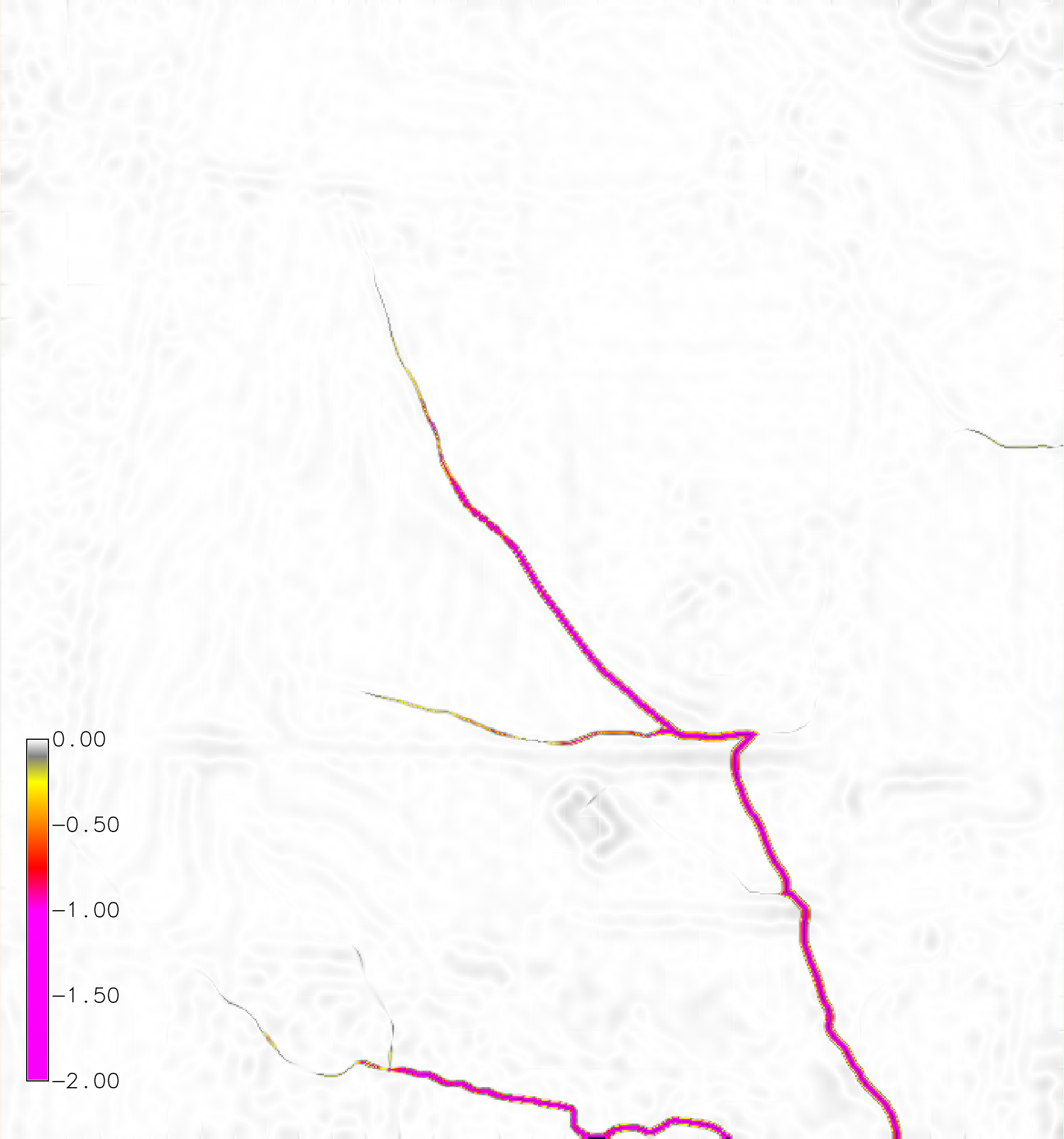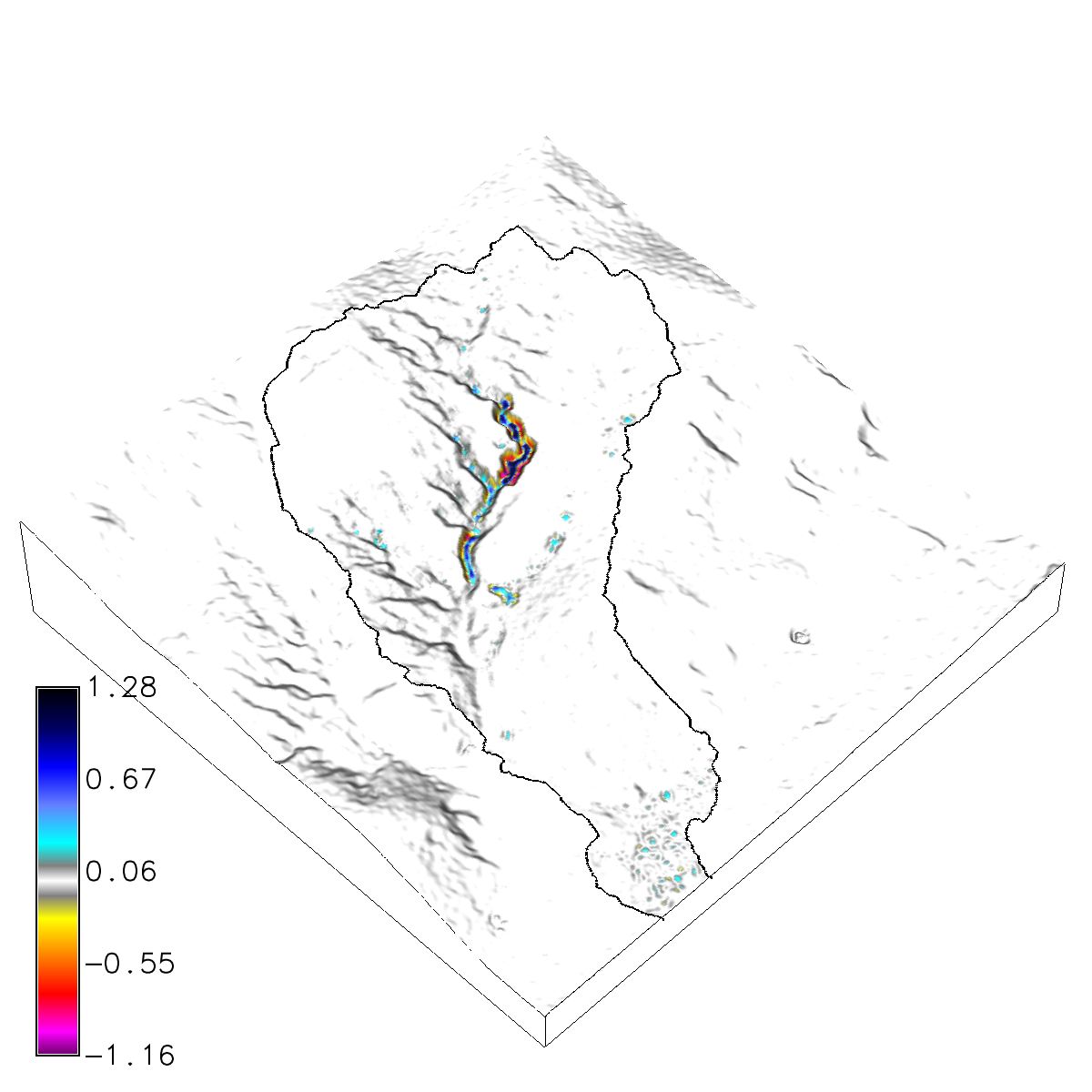## NAME

r.sim.terrain - Dynamic landscape evolution model

## KEYWORDS

raster, terrain, landscape, evolution

## SYNOPSIS

r.sim.terrain
r.sim.terrain --help
r.sim.terrain [-f] elevation=name runs=string mode=string [rain_intensity=integer] [rain_duration=integer] [precipitation=name] [k_factor=name] [k_factor_value=float] [c_factor=name] [c_factor_value=float] [m=float] [n=float] [walkers=integer] [runoff=name] [runoff_value=float] [mannings=name] [mannings_value=float] [detachment=name] [detachment_value=float] [transport=name] [transport_value=float] [shearstress=name] [shearstress_value=float] [density=name] [density_value=float] [mass=name] [mass_value=float] [grav_diffusion=float] [erdepmin=float] [erdepmax=float] start=string rain_interval=integer temporaltype=name [threads=integer] elevation_timeseries=name [depth_timeseries=name] [erdep_timeseries=name] [flux_timeseries=name] [difference_timeseries=name] [--overwrite] [--help] [--verbose] [--quiet] [--ui]

### Flags:

-f
Fill depressions
--overwrite
Allow output files to overwrite existing files
--help
Print usage summary
--verbose
Verbose module output
--quiet
Quiet module output
--ui
Force launching GUI dialog

### Parameters:

elevation=name [required]
Name of input elevation raster map
runs=string [required]
Run for a single rainfall event or a series of events
Options: event, series
Default: event
event: single rainfall event
series: series of rainfall events
mode=string [required]
SIMWE erosion deposition, USPED transport limited, or RUSLE 3D detachment limited mode
Options: simwe_mode, usped_mode, rusle_mode
Default: simwe_mode
simwe_mode: SIMWE erosion deposition mode
usped_mode: USPED transport limited mode
rusle_mode: RUSLE 3D detachment limited mode
rain_intensity=integer
Rainfall intensity in mm/hr
Default: 50
rain_duration=integer
Total duration of storm event in minutes
Default: 60
precipitation=name
Precipitation file
Name of input precipitation file
k_factor=name
K factor
Soil erodibility factor
k_factor_value=float
K factor constant
Soil erodibility constant
Default: 0.25
c_factor=name
C factor
Land cover factor
c_factor_value=float
C factor constant
Land cover constant
Default: 0.1
m=float
Water flow exponent
Water flow exponent
Default: 1.5
n=float
Slope exponent
Slope exponent
Default: 1.2
walkers=integer
Number of walkers (max = 7000000)
Default: 1000000
runoff=name
Runoff coefficient
Runoff coefficient (0.6 for bare earth, 0.35 for grass or crops, 0.5 for shrubs and trees, 0.25 for forest, 0.95 for roads)
runoff_value=float
Runoff coefficient
Runoff coefficient (0.6 for bare earth, 0.35 for grass or crops, 0.5 for shrubs and trees, 0.25 for forest, 0.95 for roads)
Default: 0.35
mannings=name
Manning's roughness coefficient
Manning's roughness coefficient
mannings_value=float
Manning's roughness coefficient
Manning's roughness coefficient
Default: 0.04
detachment=name
Detachment coefficient
Detachment coefficient
detachment_value=float
Detachment coefficient
Detachment coefficient
Default: 0.01
transport=name
Transport coefficient
Transport coefficient
transport_value=float
Transport coefficient
Transport coefficient
Default: 0.01
shearstress=name
Shear stress coefficient
Shear stress coefficient
shearstress_value=float
Shear stress coefficient
Shear stress coefficient
Default: 0.0
density=name
Sediment mass density
Sediment mass density in g/cm^3
density_value=float
Sediment mass density
Sediment mass density in g/cm^3
Default: 1.4
mass=name
Mass of sediment per unit area
Mass of sediment per unit area in kg/m^2
mass_value=float
Mass of sediment per unit area
Mass of sediment per unit area in kg/m^2
Default: 116.
grav_diffusion=float
Gravitational diffusion coefficient
Gravitational diffusion coefficient in m^2/s
Default: 0.1
erdepmin=float
Minimum values for erosion-deposition
Minimum values for erosion-deposition in kg/m^2s
Default: -0.5
erdepmax=float
Maximum values for erosion-deposition
Maximum values for erosion-deposition in kg/m^2s
Default: 0.5
start=string [required]
Start time in year-month-day hour:minute:second format
Default: 2016-01-01 00:00:00
rain_interval=integer [required]
Time interval between evolution events in minutes
Default: 1
temporaltype=name [required]
The temporal type of the space time dataset
Options: absolute, relative
Default: absolute
Default: 1
elevation_timeseries=name [required]
Name of the output space time raster dataset
Default: elevation_timeseries
depth_timeseries=name
Name of the output space time raster dataset
Default: depth_timeseries
erdep_timeseries=name
Name of the output space time raster dataset
Default: erdep_timeseries
flux_timeseries=name
Name of the output space time raster dataset
Default: flux_timeseries
difference_timeseries=name
Name of the output space time raster dataset
Default: difference_timeseries

## DESCRIPTION

r.sim.terrain is a short-term landscape evolution model that simulates topographic change for both steady state and dynamic flow regimes across a range of spatial scales. It uses empirical models (RUSLE3D & USPED) for soil erosion at watershed to regional scales and a physics-based model (SIMWE) for shallow overland water flow and soil erosion at subwatershed scales to compute short-term topographic change. This either steady state or dynamic model simulates how overland sediment mass flows reshape topography for a range of hydrologic soil erosion regimes based on topographic, land cover, soil, and rainfall parameters.

## EXAMPLES

Basic instructions

A basic example for the North Carolina sample dataset. Install the add-on module r.sim.terrain. Copy the raster elevation map elev_lid792_1m from the PERMANENT mapset to the current mapset. Set the region to this elevation map at 1 meter resolution. Run r.sim.terrain with the RUSLE model for a 120 min event with a rainfall intensity of 50 mm/hr at a 3 minute interval. Set the empirical coefficients m and n to 0.4 and 1.3 respectively. Use the `-f` flag to fill depressions in order to reduce the effect of positive feedback loops.
```g.extension  extension=r.sim.terrain
g.copy raster=elev_lid792_1m@PERMANENT,elevation
g.region raster=elev_lid792_1m res=1
r.sim.terrain -f elevation=elevation runs=event mode=rusle_mode rain_intensity=50.0 rain_duration=120 rain_interval=3 m=0.4 n=1.3
```Figure: Net difference (m) for a dynamic RUSLE simulation of a 120 min event with a rainfall intensity of 50 mm/hr with a 3 minute interval.

Spatially variable soil and landcover

Clone or download the landscape evolution sample dataset with a time series of lidar-based digital elevation models and orthoimagery for a highly eroded subwatershed of Patterson Branch Creek, Fort Bragg, NC, USA.

Run r.sim.terrain with the simwe model for a 120 min event with a rainfall intensity of 50 mm/hr. Use a transport value lower than the detachment value to trigger a transport limited erosion regime. Use the -f flag to fill depressions in order to reduce the effect of positive feedback loops.

```g.mapset -c mapset=transport location=nc_spm_evolution
g.region region=region res=1
g.copy raster=elevation_2016@PERMANENT,elevation_2016
r.sim.terrain -f elevation=elevation_2016 runs=event mode=simwe_mode \
rain_intensity=50.0 rain_interval=120 rain_duration=10 walkers=1000000 \
detachment_value=0.01 transport_value=0.0001 manning=mannings runoff=runoff
```Figure: Net difference (m) for a steady state, transport limited SIMWE simulation of a 120 min event with a rainfall intensity of 50 mm/hr.
For more detailed instructions and examples see this in-depth tutorial.

## ERROR MESSAGES

If the module fails with
```ERROR: Unable to insert dataset of type raster in the temporal database. The mapset of the dataset does not match the current mapset.
```
check that the input elevation map is in the current mapset. The input elevation map must be in the current mapset so that it can be registered in the temporal database.

## REFERENCES

r.sim.water, r.sim.sediment

## AUTHOR

Brendan A. Harmon
Louisiana State University
brendan.harmon@gmail.com

## SOURCE CODE

Available at: r.sim.terrain source code (history)

Latest change: Thu Feb 3 09:32:35 2022 in commit: f17c792f5de56c64ecfbe63ec315307872cf9d5c

© 2003-2022 GRASS Development Team, GRASS GIS 8.0.3dev Reference Manual